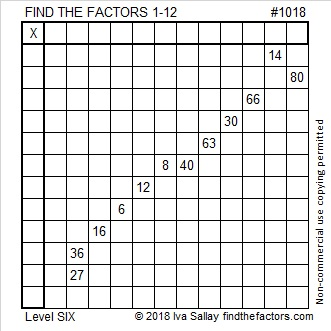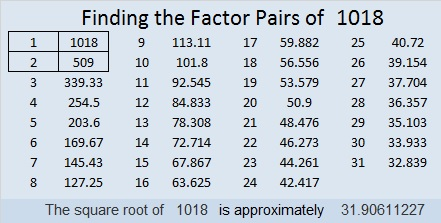# 1018 and Level 6

Level 6 puzzles can be tricky to solve, but I promise that you can still solve this one using logic and knowledge of the basic multiplication table. Just write the numbers from 1 to 12 in both the first column and the top row so that the puzzle is like a partially filled out multiplication table with the factors in a different order. Like always, there is only one solution. Can you find it?Print the puzzles or type the solution in this excel file: 12 factors 1012-1018

Look at these interesting facts about the number 1018:

27² + 17² = 1018
That means that 1018 is the hypotenuse of a Pythagorean triple:
440-918-1018 calculated from 27² – 17², 2(27)(17), 27² + 17²

It also means that (44² – 10²)/2 = 1018
Note that 27 + 17 = 44 and 27 – 17 = 10

1018 is full house 33322 in BASE 4 because 3(4⁴) + 3(4³) + 3(4²) + 2(4¹) + 2(4⁰) = 3(256 + 64 + 16) + 2(4 + 1) = 1018

Since 1018 is the sum of odd squares, it is divisible by 2. Since those odd squares have no common prime factors, you only have to check to see if 1018 is divisible by any Pythagorean triple hypotenuses less than or equal to (√1018)/2 ≈ 15.953. It is not divisible by 5 or 13, therefore 1018 only has two prime factors: 2 and 1018/2.

• 1018 is a composite number.
• Prime factorization: 1018 = 2 × 509
• The exponents in the prime factorization are 1 and 1. Adding one to each and multiplying we get (1 + 1)(1 + 1) = 2 × 2 = 4. Therefore 1018 has exactly 4 factors.
• Factors of 1018: 1, 2, 509, 1018
• Factor pairs: 1018 = 1 × 1018 or 2 × 509
• 1018 has no square factors that allow its square root to be simplified. √1018 ≈ 31.90611This site uses Akismet to reduce spam. Learn how your comment data is processed.